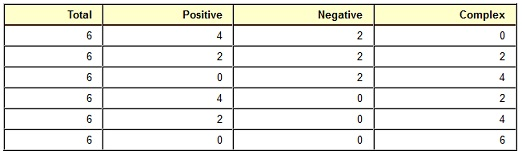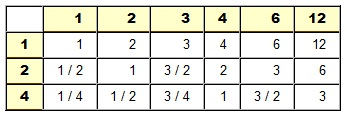#### Theory of Real Zeros of Polynomial Functions

Real Zeros of Polynomial Functions:

Long Division of Polynomials:

We can know long division of polynomials in Intermediate Algebra. Fundamentally, the procedure is taken out like long division of the real numbers.

One of the key point regarding division, and this works for real numbers and also for polynomial division, requires to be pointed out. Whenever you divide the dividend by divisor, you obtain a quotient and a remainder. To ensure the problem, you multiply the divisor by the quotient and add up the remainder to obtain the dividend. When the remainder is 0, then we state that the divisor divides equally to the dividend.

Dividend/Divisor = Quotient + Remainder/Divisor

Dividend = Divisor * Quotient + Remainder

The similar thing can be completed with polynomial functions.

f(x) = d(x) * q(x) + r(x)

Where f(x) is a polynomial function being divided to dividend, d(x) is the polynomial function being divided by the divisor, q(x) is the polynomial function which is the quotient, and r(x) is the polynomial remainder function and will contain degree less than divisor.

When the remainder r(x), is zero, then f(x) = d(x)*q(x). We have simply factored the function f(x) into the two factors, d(x) and q(x).

Remainder Theorem:

Whenever a polynomial function f is divided by the x-k, then the remainder r is f(k).

In English, whenever you divide a polynomial by the linear factor, x-k, then the remainder is a value you would get when you plugged x = k to the function and computed.

Now, tie that to what we just state above. When the remainder is zero, then you encompass successfully factored the polynomial. When the remainder if dividing by (x-k) is zero, then the function computed at x = k is zero and you found a zero or root of the polynomial. In addition, you now have a factored polynomial (that is, the quotient) that is one less degree than the original polynomial. When the quotient is down to the quadratic or linear factor, then you can resolve and find out the other solutions.

Synthetic Division:

To divide a polynomial synthetically by x-k, execute the given steps.

Setup:

a) Write k down, leave a few space after it.

b) On similar line, right the coefficients of polynomial function. Make sure that you write the coefficients in order of reducing power. Be sure to place a zero down when a power is missing. Place holders are extremely important.

c) For now, leave a blank line. Draw left and bottom parts of a box. The left part goes between the k and coefficients. The bottom part goes beneath the blank line you left.

Synthetic Division:

Once you include things set up, you can really start to execute the synthetic division.

a) Bring the very first coefficient down to the bottom row (beneath the line).

b) Multiply the number in bottom row by the constant k, and write down the product in the next column of second row (that is, above the line).

c) Add the numbers in next column and write down the total beneath the line.

d) Repeat the steps 2 and 3 till all the columns are filled up.

Interpreting the Results:

a) The very last value is remainder. When the remainder is zero, you have found a zero of function.

b) The rest of values are the coefficients of quotient. Each and every term will be increased to the one less power than original dividend. (When it was a fourth degree polynomial to begin with, the quotient will be a third degree polynomial).

Note: You can only utilize synthetic division as explained above to divide by x-k. That is, it should be a linear factor, and the leading coefficient should be one.

There are similar manners to divide by a quadratic, cubic, and so on however for certain reason, they are not taught anymore.

Fundamental Theorem of Algebra:

Each and every polynomial in one variable of degree n, n > 0, has at least one real or complex zero.

Influence to the Fundamental Theorem of Algebra:

Each and every polynomial in one variable of degree n, n > 0, has precisely n real or complex zeros.

Complex Roots:

The Complex solutions come in pairs. If (a+bi) is the solution, then its complex conjugate (a-bi) is as well a solution.

Square Roots:

Solutions comprising square roots as well come in pairs. If a+sqrt(b) is a solution, then its conjugate, a-sqrt(b) will as well be a solution. The similar is not essentially true of other roots.

Descartes' Rule of Signs:

a) The maximum number of positive real roots can be determined by counting the number of sign changes in the f(x). The real number of positive real roots might be the maximum, or the maximum reduced by a multiple of two.

b) The maximum number of negative real roots can be determine by counting the number of sign changes in the f(-x). The real number of negative real roots might be the maximum, or the maximum reduced by a multiple of two.

c) Complex roots forever come in pairs. That is why the number of positive or negative roots should decrease by two.

Let consider: f(x) = 3x^6 + x^5 - x^4 + 3x^3 + 2x^2 - x + 1.

The signs in f(x) are + + - + + - +. There are 4 sign modifications (+ to -) or (- to +).

Now, f(-x) = 3x^6 - x^5 - x^4 - 3x^3 + 2x^2 + x + 1.

The signs in f(-x) are + - - - + + +. There are 2 sign modifications (+ to -) or (- to +).

The Possible Combinations of Roots are as follows:Note that the negative and positive values can reduce by two independently of one other.

Rational Root Test:

When a polynomial function consists of integer coefficients, then each and every rational zero will encompass the form p/q where p is the factor of the constant and q is a factor of leading coefficient.

a) Make sure that the polynomial consists of integer coefficients. Multiply to get rid of decimals or fractions if need be (be sure to afterward divide).

b) This just addresses the rational zeros. There might be real, however non-rational roots. There might be complex roots comprising i.

c) This states that the rational zeros will encompass this form. It doesn’t state that everything which has this form is a rational zero. What it does provide you is a list of possible rational zeros.

Illustration: f(x) = 4x^5 - x^2 + 12

The Possible rational zeros will be of the form (that is, factor of 12) over (the factor of 4). The division table can aid you in finding all these values.The division table helps in identifying the possible rational zeros. You must throw out the duplicates, and list others in order.

-12, -6, -4, -3, -2, -3 / 2, -1, -3 / 4, -1 / 2, -1 / 4, 1 / 4, 3 / 4, 1, 3 / 2, 2, 3, 4, 6, 12

Now, you execute synthetic division on possible rational zeros till you find out one.

Here is where the Descartes' Rule of Signs comes in. In this specific problem, there would be a maximum of 2 positive and 1 negative root. That signifies that you might contain 2 or 0 positive roots, however you will always have 1 negative. There is no guarantee that the negative is rational, although. Descartes just guaranteed real roots. When there were no negatives, then you would know not to try any.

Upper and Lower Bounds:

When you have a polynomial with real coefficients and with positive leading coefficient, then...

Upper Bound:

When synthetic division is executed by dividing by x-k, where k>0, and all the signs in bottom row of synthetic division are non-negative, then x = k is the upper bound (nothing is bigger) for the zeros of polynomial.

Lower Bound:

When synthetic division is executed by dividing by x-k, where k<0, and the signs in bottom row of synthetic division alternate (among non-negative and non-positive), then x = k is a lower bound (nothing is minimum) for the zeros of polynomial.

The zero in the bottom row might be considered positive or negative as required.

Suggested Attack to Finding Zeros of a Polynomial:

a) Recognize the total number of real or complex zeros (effect to Fundamental Theorem of Algebra).

b) Recognize the possible number of positive, negative and complex zeros (that is, Descartes' Rule of Signs).

c) List all the possible rational zeros (that is, Rational Root Theorem)

d) Try possible rational zeros till you find out one that works. Subsequent to each division by a positive value, ensure for possible upper bounds. After each and every division by a negative value, ensure for possible lower bounds (that is, Upper and Lower Bound Theorems)

e) After you find out a possible rational root which actually works, take the quotient and carry on to try to factor it till it is down to quadratic or less. Once it is a quadratic or less, then there are other ways to resolve it.

f) Write linear and or linear/irreducible quadratic factorization.

Really significant (and annoying if you forget)!

Once you have found a zero by using synthetic division, utilize the quotient as a new polynomial for all the further divisions. The quotient will be one less degree than original dividend. Each and every time you find out a root, the quotient becomes one less in degree. Ultimately, it will become a quadratic, and then you can factor, extract roots, complete the square, and use the quadratic equation to find out the remaining roots.

If you carry on to utilize the original function, you will become much frustrated and waste a lot of time.

Latest technology based Algebra Online Tutoring Assistance

Tutors, at the www.tutorsglobe.com, take pledge to provide full satisfaction and assurance in Algebra help via online tutoring. Students are getting 100% satisfaction by online tutors across the globe. Here you can get homework help for Algebra, project ideas and tutorials. We provide email based Algebra help. You can join us to ask queries 24x7 with live, experienced and qualified online tutors specialized in Algebra. Through Online Tutoring, you would be able to complete your homework or assignments at your home. Tutors at the TutorsGlobe are committed to provide the best quality online tutoring assistance for Algebra Homework help and assignment help services. They use their experience, as they have solved thousands of the Algebra assignments, which may help you to solve your complex issues of Algebra. TutorsGlobe assure for the best quality compliance to your homework. Compromise with quality is not in our dictionary. If we feel that we are not able to provide the homework help as per the deadline or given instruction by the student, we refund the money of the student without any delay.